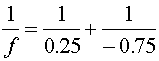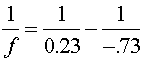Examples for optical instruments

Example #1

Problem:

a.) Grandpa's far point is 75 cm. What is the prescription (refractive power in diopters) for Grandpa's contact lenses?

Solution:

A diverging lens will make an image of a far away object at its focal length. Therefore, the focal length should be negative 75 cm, and the prescription is

-1.33 diopters

b.) What is his prescription (in diopters) for eye glasses?

Solution:

Allowing for 2 cm between the eye and the lens of the glasses, the image must be brought to 73 cm. Therefore the prescription is

-1.37 diopters

c.) Grandma's near point is 75 cm. What is the prescription (in diopters) for Grandma's contact lenses?

Solution:

Choose the focal length such that an object at the normal near point of 25 cm produces an image where Grandma can focus on it, at 75 cm., and the prescription is

2.67 diopters

d.) What is her prescription for eye glasses?

Solution:

Allowing for 2 cm between the eye and the glasses, the normal near point is 23 cm from the glasses and her near point is 73 cm from the lenses,.

2.81 diopters

Example #2

Problem:

a.) A simple two-lens telescope's eyepiece has a focal length of 10 cm. If you want the magnification of the telescope to be 40, what is the length of the telescope?

Solution:

The second lens must have a focal length 40 times longer, 400 cm. The overall length is thus

4.10 m